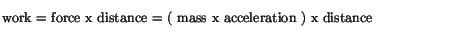Next: Potential Energy Up: Work and Energy Previous: Power

# Kinetic Energy

The work done on an object involves the force exerted on it, which by Newton's 2nd law can be related to the acceleration produced. Let us use this in the definition of work:The acceleration in turn implies a change in velocity. One defines a quantity called the kinetic energy of an object aswith which one can show thatKinetic energy (and as we shall later see, all forms of energy'') has the units of work, which are Joules. Thus, the work done on any object goes into changing the kinetic energy of that object.

As an example, we can calculate the power of a 1000 kg sports car that can accelerate from 0 to 100 km/hr in 10 seconds. In order to be able to get our answer directly in Joules we first convert the speed to m/s.Since the work done by the car engine is equal to the change in kinetic energy of the car, we have thatThe corresponding power in watts is obtained by dividing the work done in Joules by elapsed time, so that the power is 386,000/10 = 38,600 watts. In terms of horsepower, we see that the car has 38,600/746 = 53 hp. In a real car, much of the work done by the engine goes into overcoming friction and air resistance, so that a 53 hp engine will not produce the type of acceleration mentioned in this idealized example.

Now that we know that work changes kinetic energy, it is possible to give another interpretation to the notion of negative work. Positive work done on an object increases its kinetic energy, as stated above, whereas negative work will decrease its kinetic energy. This is also made clear by our previous example of negative work: if you push against a car that is rolling towards you in order to slow it down, you are indeed decreasing its kinetic energy.Next: Potential Energy Up: Work and Energy Previous: Power
modtech@theory.uwinnipeg.ca
1999-09-29